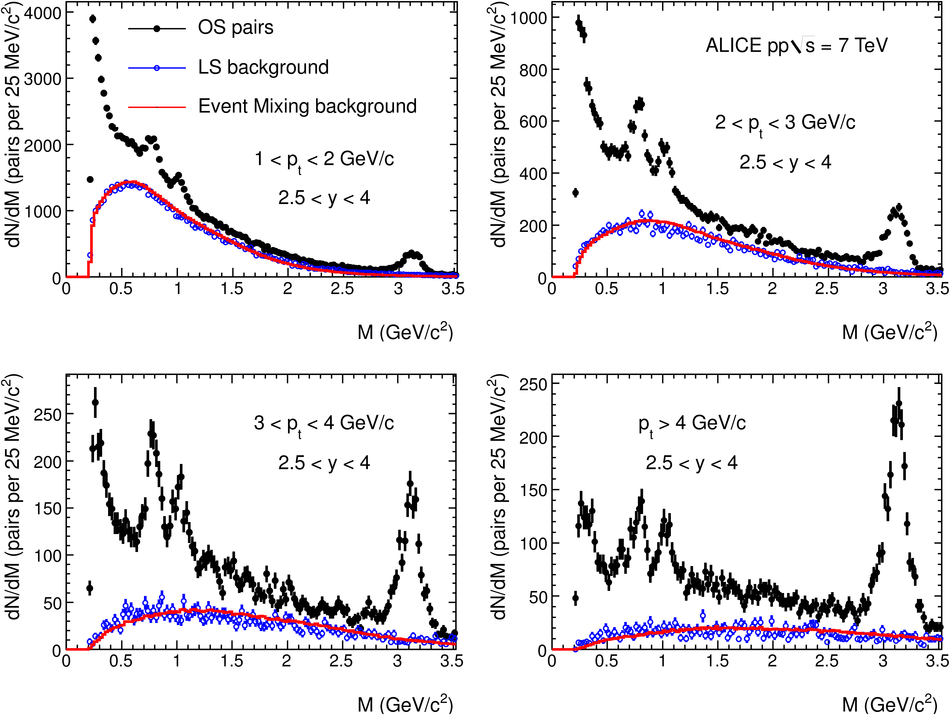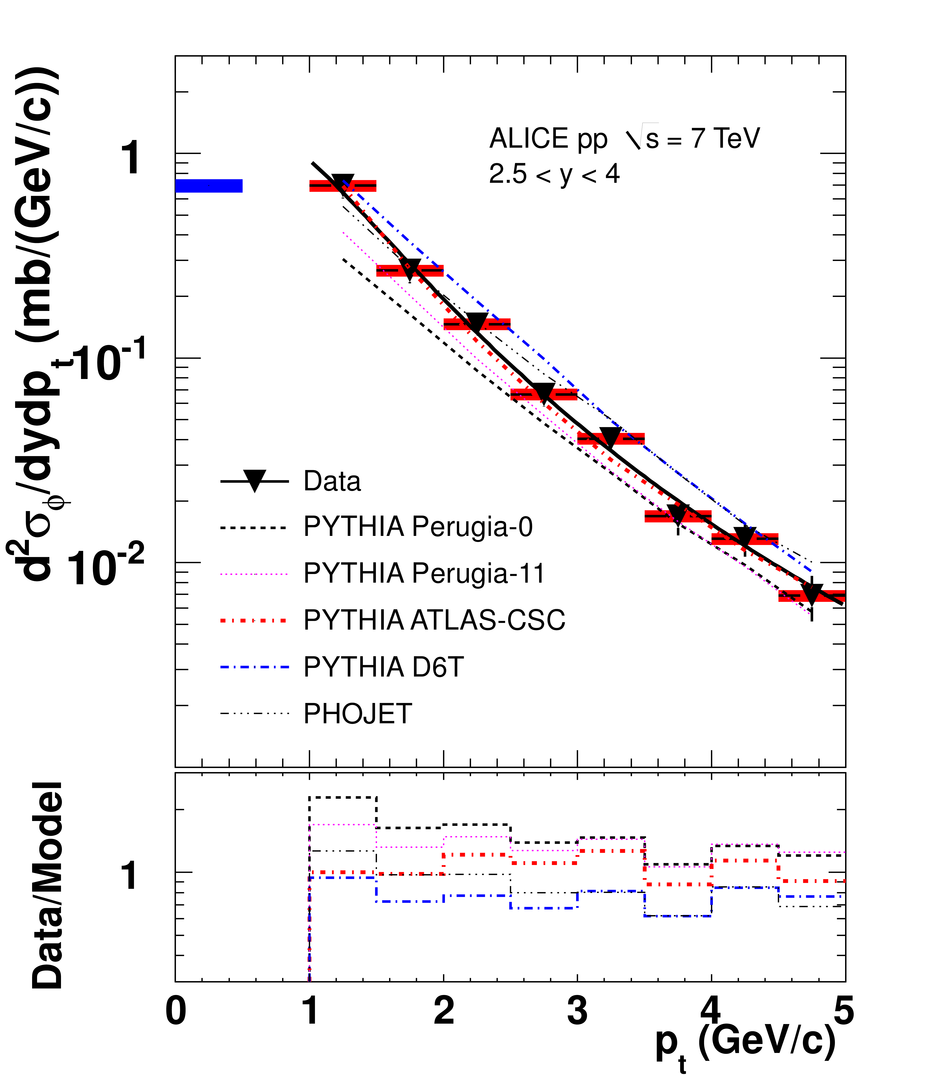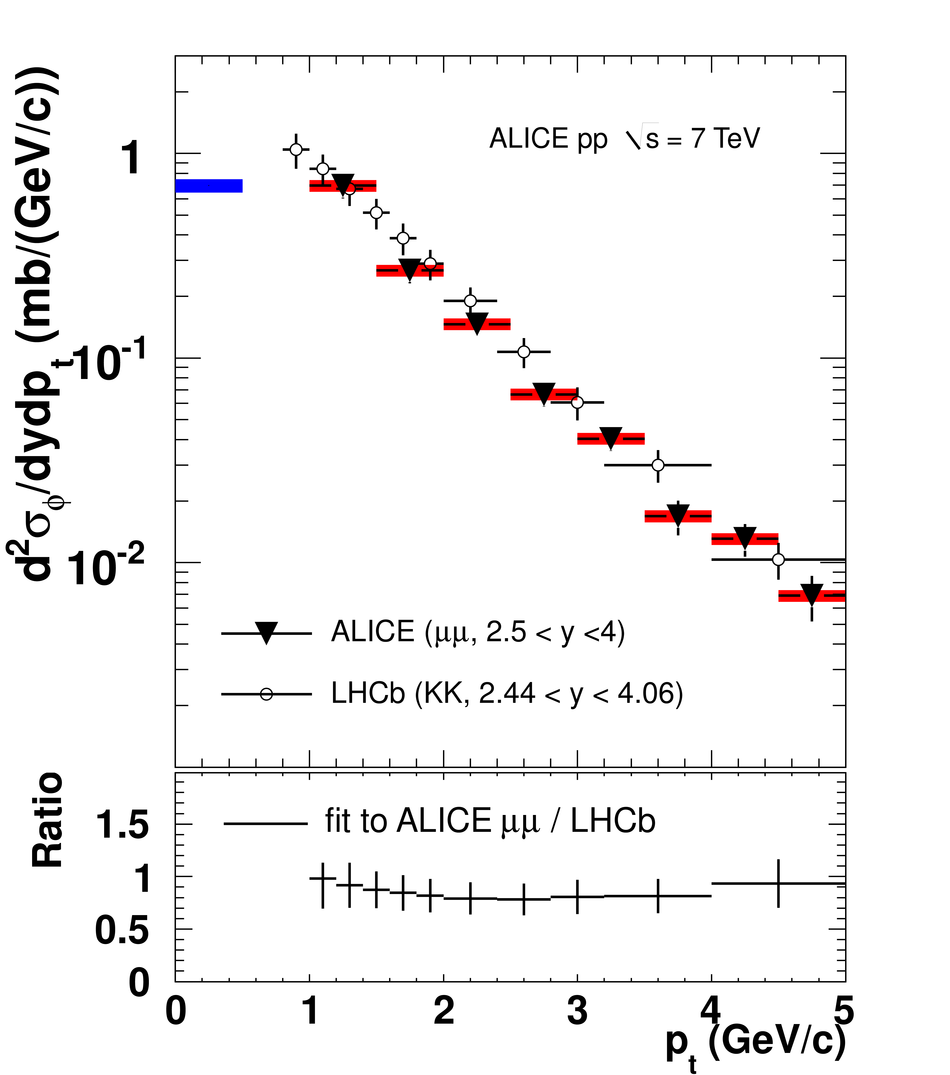# Light vector meson production in pp collisions at $\sqrt{s}$ = 7 TeV

The ALICE experiment has measured low-mass dimuon production in pp collisions at $\sqrt{s} = 7$ TeV in the dimuon rapidity region 2.5<~y<~4. The observed dimuon mass spectrum is described as a superposition of resonance decays ($\eta$, $\rho$, $\omega$, $\eta^{'}$, $\phi$) into muons and semi-leptonic decays of charmed mesons. The measured production cross sections for $\omega$ and $\phi$ are $\sigma_\omega$ (1<~$p_{\rm T}$<~5 GeV/$c$,2.5<~y<~4) = 5.28 $\pm$ 0.54 (stat) $\pm$ 0.50 (syst) mb and $\sigma_\phi$(1<~$p_{\rm T}$<~5 GeV/$c$,2.5<~y<~4)=0.940 $\pm$ 0.084 (stat) $\pm$ 0.078 (syst) mb. The differential cross sections $d^2\sigma/dy dp_{\rm T}$ are extracted as a function of $p_{\rm T}$ for $\omega$ and $\phi$. The ratio between the $\rho$ and $\omega$ cross section is obtained. Results for the $\phi$ are compared with other measurements at the same energy and with predictions by models.

Figures

## Figure 1

 Invariant mass spectra for opposite-sign muon pairs in pp at $\sqrt{s}=7$~TeV in different $\pt$ ranges The combinatorial background, evaluated from opposite-sign pairs in mixed events (red line) or like-sign pairs in non-mixed events (blue points), is also shown.## Figure 2

 Dimuon invariant mass spectrum in pp at $\sqrt{s}=7$~TeV after combinatorial background subtraction for $\pt>1$~GeV$/c$ (triangles) Light blue band: systematic uncertainty from background subtraction Red band: sum of all simulated contributions. The width of the red band represents the uncertainty on the relative normalization of the sources## Figure 3

 Top: Inclusive differential $\phi$ production cross section${\rm d}^2\sigma_\phi/{\rm d}y {\rm d}\pt$ for $2.5 < y < 4$ The error bars represent the quadratic sum of the statistical and systematic uncertainties, the red boxes the point-to-point uncorrelated systematic uncertainty, the blue box ont he left the error on normalization. Data are fitted with (Eq.1)(solid line) and compared with the Perugia-0, Perugia-11,ATLAS-CSC and D6T PYTHIA tunes and with PHOJET Bottom: Ratio between data and models. \begin{align*} &(1)& dN \over dp_{\rm t} &= C&p_{\rm t} \over [1 +(p_{\rm t}/p_{\rm 0})^2]^n \end{align*}## Figure 4

 Top: Inclusive differential $\phi$ production cross section${\rm d}^2\sigma_\phi/{\rm d}y {\rm d}\pt$, as measured via the decayinto dimuons (black triangles). The blue box onthe left represents the error on normalization The data are compared to the measurementsin the kaon decay channel by LHCb (black open circles)~ Bottom: Fit to the differential cross section measured in dimuonsdivided by the cross section measured in the kaon channel by LHCb## Figure 5

 Ratio $N_\phi /(N_\rho + N_\omega)$ as a function of the dimuon transverse momentum.## Figure 6

 Top: Inclusive differential $\omega$ production cross section${\rm d}^2\sigma_\omega/{\rm d}y {\rm d}\pt$ for $2.5 < y < 4$ The error bars represent the quadratic sum of the statistical and systematicuncertainties, the red boxes thepoint-to-point uncorrelated systematic uncertainty, the blue box onthe left the error on normalization. Data are fitted with Eq.~(1)(solid line) and compared with the Perugia-0, Perugia-11,ATLAS-CSC and D6T PYTHIA tunes and PHOJET Bottom: Ratio between data and models. \begin{align*} &(1)& dN \over dp_{\rm t} &= C&p_{\rm t} \over [1 +(p_{\rm t}/p_{\rm 0})^2]^n \end{align*}